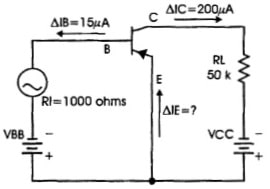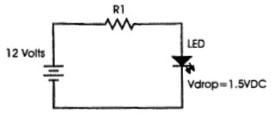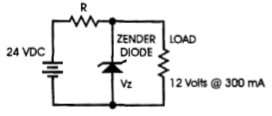# Circuit Assignment Question

1. A power supply produces 24 volts at full load with a percent regulation of 10%, calculate the no-load voltage of the supply.

2. A common-emitter transistor amplifier has a base current change of 15 microamperes and a collector current change of 200 microamperes. The load resistance is 50000 ohms with an input resistance of 1000 ohms.Calculate the AC alpha, AC beta, AC voltage gain and power gain of the above circuit.

3. A series-tuned resonant circuit operates at a resonant frequency of 1 MHz. It contains a .01 'LF capacitor and a 300 'LH inductor with 20 ohms of resistance. Calculate the circuit's quality factor and bandwidth at its resonant frequency.

4. You must design a series-tuned LC circuit with a resonant frequency of 2 kHz. The circuit Wiil include a 2 mH inductor. What size capacitor should you install?

5. In the circuit below the LED's rated current is 20 millamperes. With a voltage drop across the LED of 1.5 volts, calculate the value of the series resistance RI necessary to be used if the supply voltage is 12 volts DC.6. In the zener diode regulator circuit below calculate the value of the current through the zener Diode if the power supply is rated at 24 volts @ 500 milliamperes. The load voltage is 12 volts and draws a current of 300 milliamperes. Aso calculate the value of the series resistance R.